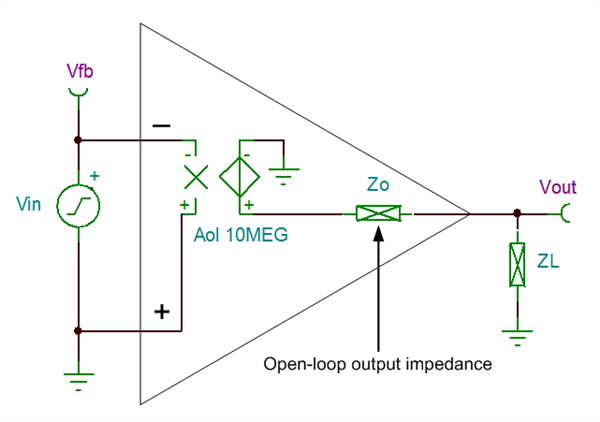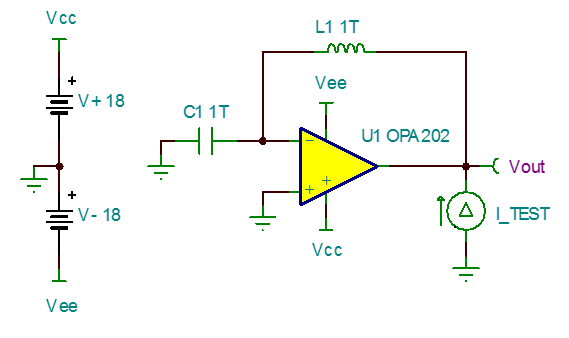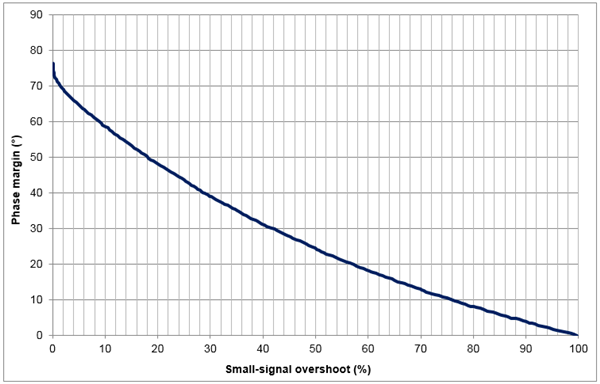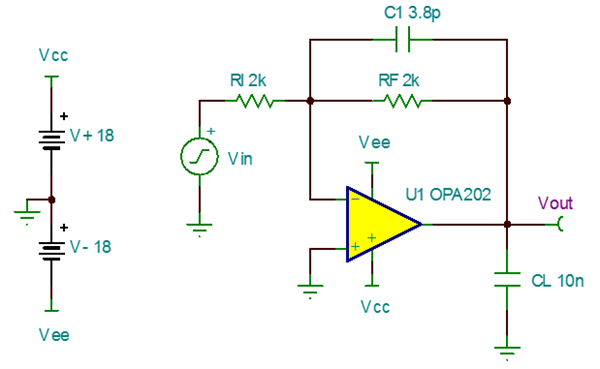# “Trust, but verify” SPICE model accuracy, part 4: open-loop output impedance and small-signal overshoot

Other Parts Discussed in Post: OPA202

Previous installments of this blog post series discussed the need for verifying SPICE model accuracy and showed how to measure common-mode rejection ratio (CMRR), offset voltage versus common-mode voltage (Vos vs. Vcm) and slew rate (SR). In part four, I’ll continue putting operational amplifier (op amp) SPICE models to the test by checking their usefulness for small-signal stability analysis. Whether instability rears its ugly head as overshoot and ringing, continuous oscillation, or other more bizarre behavior, it can prove to be a real beast to debug.

Thankfully, an accurate SPICE model is a valuable asset in the struggle to solve op amp stability issues. A good model, combined with the powerful analysis tools available in simulation, can help predict and stabilize op amp circuits before they get a chance to cause trouble in the real world.

While many different stability compensation methods exist, a thorough discussion of stability compensation is beyond the scope of this post. Instead, I’ll focus on how to verify that an op amp SPICE model is accurate for use in stability analysis by comparing model performance versus the data sheet. If you wish to dive deeper into op amp stability theory and compensation techniques, start by watching our TI Precision Labs – Op Amps video series on stability.

Open-loop output impedance

The most critical specification to check for accuracy before performing stability analysis is the op amp’s open-loop output impedance, or Zo. At a basic level, you can think of Zo as a complex impedance in the op amp’s small-signal path, which occurs between the open-loop gain stage (Aol) and the output pin. This impedance interacts with the op amp’s Aol, as well as any load and feedback present, to create the circuit’s overall AC response. Figure 1 is a simplified schematic-level view of Zo in an open-loop op amp circuit.Figure 1: Simplified op amp small-signal model

If op amp manufacturers do not model Zo accurately, then the overall small-signal AC behavior of an op amp model is incorrect and can’t be used for stability analysis. Thankfully, it’s easy to verify that a model’s Zo matches the data sheet. Figure 2 shows the recommended test circuit.Figure 2: Open-loop output impedance test circuit

In this test circuit, inductor L1 creates closed-loop feedback at DC while allowing for open-loop AC analysis, and capacitor C1 shorts the inverting input to ground at AC to prevent the node from floating. AC current source I_TEST back-drives the op amp output, and by measuring the resulting voltage at the output pin, you can determine the output impedance using Ohm’s law.

To plot Zo, run an AC transfer function over the desired frequency range and plot the voltage at Vout. Note that many simulators default to showing the results in decibels. If you plot the measurement on a logarithmic scale, Vout is equivalent to ohms. Let’s now test the Zo of the OPA202, a new precision bipolar amplifier from TI.

Figure 3: OPA202 Zo results

In this case, the op amp’s Zo is modeled very closely to the data-sheet spec. The output impedance is also very flat (that is, resistive) up to around 1MHz, typical of classic bipolar amplifier designs. Confident that the model’s Zo is correct, let’s now check the rest of the small-signal response.

Small-signal overshoot

One of the simplest ways to check for op amp stability (both with simulation and in the real world) is to measure the percent overshoot at the output in response to a step or square-wave input. Assuming that the op amp circuit is a second-order system, overshoot can be related to phase margin (and therefore stability) based on their mathematical relationship to each other through the damping factor. Figure 4, taken from the “Analog Engineer’s Pocket Reference,” shows this relationship as overshoot increases from zero to 100%.Figure 4: Phase margin vs. percent overshoot

You can test small-signal overshoot in both inverting and noninverting configurations, but today I’ll be demonstrating the inverting configuration shown in Figure 5. RF and RI are set to the op amp’s typical load resistance of 2kΩ and configure the closed-loop gain to -1V/V. CF provides compensation of the op amp input capacitance and is set equal to C_CM + C_DIFF, while capacitive load CL is set to 10nF. Vin generates a 5mVpk square wave at 10kHz, ensuring that the op amp shows only small-signal behavior.Figure 5: Small-signal step response test circuit, gain = -1V/V

Let’s use this circuit to measure the small-signal overshoot of the OPA202. To do this, first run a transient analysis over one period, or 100µs, and plot the voltage at Vin and –(Vout). Since this is an inverting amplifier setup, I recommend inverting the output waveform for easier comparison against the input.

Figure 6: OPA202 small-signal overshoot, gain = -1V/V, CL = 10nF

Equations 1 and 2 calculate the percent overshoot:

% overshoot = 100 * [(Vmax – Vfinal) / Vstep]                 (1)

% overshoot = 100 * [(7.11 mV – 5 mV) / 10 mV] = 21.1 %                      (2)

where Vmax is the maximum output voltage, Vfinal is the final settled output voltage and Vstep is the total output step size.

Referring back to the chart in Figure 4, a percent overshoot of 21.1% corresponds to roughly 47 degrees of phase margin. One general recommendation for stability is that a circuit should have at least 45 degrees of phase margin, so this just meets that requirement. It’s quite remarkable that the OPA202 is still stable even with a 10nF load!

You can repeat this test with different capacitive loads to see how well the OPA202 model matches the data-sheet capacitive load drive spec. Figure 7 gives those results.

Figure 7: OPA202 overshoot vs. capacitive load comparison

Sweeping CL from approximately 30pF to 25nF, the SPICE model overshoot aligns quite closely with the data-sheet curve, especially at heavier loads. This indicates that the small-signal characteristics of the SPICE model very closely match the real device, and any stability compensation done with simulation will translate well to the real world.

Thanks for reading this fourth installment of the “Trust, but verify” blog series! In the next installment, I’ll discuss how to measure open loop gain (AOL) and input offset voltage (VOS). If you have any questions about simulation verification, log in and leave a comment, or visit the TI E2E™ Community Simulation Models forum.